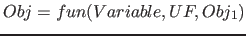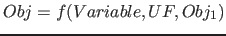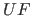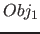Next: Numbers and variables as Up: Elements of the Fib Previous: Area element   Contents   Index

## Functions

Functions are Fib elements that assign to the variable, that they define, a value, that is calculated using a formula. For that a function contains a subfunction. A subfunction is a number, variable, or a true subfunction.

Syntax:(An alternativ for "fun" is "fkt".)

Short syntax:Description of the elements:

•: The variable, which the function element defines.
•: This is the subfunction of the function.
•: The subobject, for which theis defined and which will be evalued for the calculated variable assignment of the function.

Subsections

Betti Österholz 2013-02-13